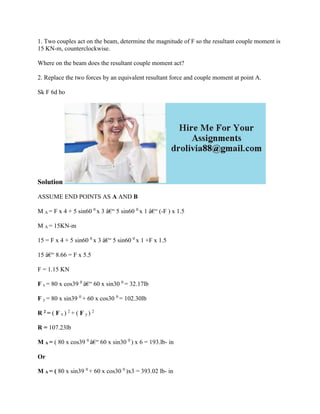Ce diaporama a bien été signalé.
Le téléchargement de votre SlideShare est en cours. ×

# 1- Two couples act on the beam- determine the magnitude of F so the re.docx

Publicité
Publicité
Publicité
Publicité
Publicité
Publicité
Publicité
Publicité
Publicité
Publicité
PublicitéChargement dans…3
×

## Consultez-les par la suite

1 sur 2 Publicité

# 1- Two couples act on the beam- determine the magnitude of F so the re.docx

1. Two couples act on the beam, determine the magnitude of F so the resultant couple moment is 15 KN-m, counterclockwise.
Where on the beam does the resultant couple moment act?
2. Replace the two forces by an equivalent resultant force and couple moment at point A.
Sk F 6d bo
Solution
ASSUME END POINTS AS A AND B
M A = F x 4 + 5 sin60 0 x 3 â€“ 5 sin60 0 x 1 â€“ (-F ) x 1.5
M A = 15KN-m
15 = F x 4 + 5 sin60 0 x 3 â€“ 5 sin60 0 x 1 +F x 1.5
15 â€“ 8.66 = F x 5.5
F = 1.15 KN
F x = 80 x cos39 0 â€“ 60 x sin30 0 = 32.17lb
F y = 80 x sin39 0 + 60 x cos30 0 = 102.30lb
R 2 = ( F x ) 2 + ( F y ) 2
R = 107.23lb
M A = ( 80 x cos39 0 â€“ 60 x sin30 0 ) x 6 = 193.lb- in
Or
M A = ( 80 x sin39 0 + 60 x cos30 0 )x3 = 393.02 lb- in
.

1. Two couples act on the beam, determine the magnitude of F so the resultant couple moment is 15 KN-m, counterclockwise.
Where on the beam does the resultant couple moment act?
2. Replace the two forces by an equivalent resultant force and couple moment at point A.
Sk F 6d bo
Solution
ASSUME END POINTS AS A AND B
M A = F x 4 + 5 sin60 0 x 3 â€“ 5 sin60 0 x 1 â€“ (-F ) x 1.5
M A = 15KN-m
15 = F x 4 + 5 sin60 0 x 3 â€“ 5 sin60 0 x 1 +F x 1.5
15 â€“ 8.66 = F x 5.5
F = 1.15 KN
F x = 80 x cos39 0 â€“ 60 x sin30 0 = 32.17lb
F y = 80 x sin39 0 + 60 x cos30 0 = 102.30lb
R 2 = ( F x ) 2 + ( F y ) 2
R = 107.23lb
M A = ( 80 x cos39 0 â€“ 60 x sin30 0 ) x 6 = 193.lb- in
Or
M A = ( 80 x sin39 0 + 60 x cos30 0 )x3 = 393.02 lb- in
.

Publicité
Publicité

### 1- Two couples act on the beam- determine the magnitude of F so the re.docx

1. 1. 1. Two couples act on the beam, determine the magnitude of F so the resultant couple moment is 15 KN-m, counterclockwise. Where on the beam does the resultant couple moment act? 2. Replace the two forces by an equivalent resultant force and couple moment at point A. Sk F 6d bo Solution ASSUME END POINTS AS A AND B M A = F x 4 + 5 sin60 0 x 3 â€“ 5 sin60 0 x 1 â€“ (-F ) x 1.5 M A = 15KN-m 15 = F x 4 + 5 sin60 0 x 3 â€“ 5 sin60 0 x 1 +F x 1.5 15 â€“ 8.66 = F x 5.5 F = 1.15 KN F x = 80 x cos39 0 â€“ 60 x sin30 0 = 32.17lb F y = 80 x sin39 0 + 60 x cos30 0 = 102.30lb R 2 = ( F x ) 2 + ( F y ) 2 R = 107.23lb M A = ( 80 x cos39 0 â€“ 60 x sin30 0 ) x 6 = 193.lb- in Or M A = ( 80 x sin39 0 + 60 x cos30 0 )x3 = 393.02 lb- in